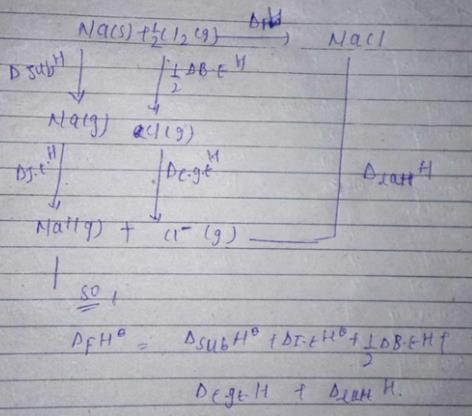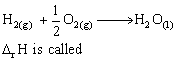Courses

# Test: Enthalpy Change

## 10 Questions MCQ Test Chemistry for JEE | Test: Enthalpy Change

Description
This mock test of Test: Enthalpy Change for JEE helps you for every JEE entrance exam. This contains 10 Multiple Choice Questions for JEE Test: Enthalpy Change (mcq) to study with solutions a complete question bank. The solved questions answers in this Test: Enthalpy Change quiz give you a good mix of easy questions and tough questions. JEE students definitely take this Test: Enthalpy Change exercise for a better result in the exam. You can find other Test: Enthalpy Change extra questions, long questions & short questions for JEE on EduRev as well by searching above.
QUESTION: 1

### Among the following enthalpies, which is always less than zero?

Solution:

The symbol represents enthalpy of combustion which is always exothermic. For exothermic rxn delta H is always -ve.

QUESTION: 2

### Enthalpy change for a cyclic process is

Solution:

Enthalpy is a state function; which means change in enthalpy depends only on final and initial state. So, the change in enthalpy for a cyclic process is zero.

QUESTION: 3

### The solubility of fluorides is much less as compared to corresponding chlorides

Solution:

Due to high lattice enthalpy of fluorides, it is difficult to break the lattice of fluoride compounds(due to the small size of fluorine, so the lattice is strong). SO the solubility of fluorides is less than chlorides.

QUESTION: 4

The enthalpy change when 1 mole of it dissolves in a specified amount of solvent is known as enthalpy of

Solution:

Enthalpy of solution (ΔHsoln) is the change in enthalpy that occurs when a specified amount of solute dissolves in a given quantity of solvent.

QUESTION: 5

The increasing order of enthalpy of vaporization of NH3, PH3, and AsH3 is

Solution:

The interparticle forces are strongest in NH3 (Hydrogen bonding). In PH3 and AsH3 the inter-particle forces are dipole-dipole forces which are relatively stronger in AsH3(due to higher mass of AsH3). So the increasing order of vaporization is PH3<AsH3<NH3 .

QUESTION: 6

The enthalpy of formation of NaCl can be calculated by using

Solution:QUESTION: 7

In Born-Haber cycle, the sum of the enthalpy changes around a cycle is

Solution:

The Born-Haber cycle is based on Hess Law or Law of constant heat summation. It states that the standard enthalpy of reaction is the sum of algebraic sum of the standard enthalpies of reactions into which the overall reaction may be splitted. So the change in enthalpy around a cycle is zero.

QUESTION: 8

The enthalpies of fusion for all reactions are

Solution:

The enthalpies of fusion for all reactions are always positive because melting of a solid is an endothermic process in which heat is supplied to the reaction Hence the sum of enthalpies of the product is greater than the sum of enthalpies of the reactant.

QUESTION: 9

In the reaction:Solution:

∆Hr is called both enthalpy of combustion of hydrogen as well as enthalpy of formation of water.For the enthalpy of combustion of hydrogen, the reason is that 1 mole of Hydrogen is burnt in its pure state. For the formation of water, the reason is that 1 mole of water is formed from its constituent elements.

QUESTION: 10

What happens to the ions, when an ionic compound dissolves in a solvent?

Solution:

When an ionic compound is dissolved in the solvent, the compound dissociate into ions and due to external medium i. e. solvent it leave its original position in crystal lattice n gets hydrated..
Eg. NaCl(aq) → Na+(aq) + Cl-(aq)